# Breast Tumor Classification Using FFT based Fractal Analysis B.MONICA JENEFER V.CYRILRAJ   Abstract Breast cancer is formed by abnormal cells, it causes fast death among human and it is shapeless. The growth of the cancer is also fast and it should be removed from the earlier stage itself. In this study, we have introduced and implemented an FFT based fractal model to analyze the breast tumor and classify it as benign or malignant according to their shapes. The benign and malignant are different in contour and shape where benign have a smooth contour and macrolobulated shapes and malignant have rough contour and irregular shapes. In this study, the contours are classified using fractal based Fourier transform method. The magnitude and frequency based features are utilized for classification. This approach achieved 92% of accuracy in tumor classification using fractal based fourier transform. Keywords: Fractal Analysis, Breast Cancer, Background study A fractal is a mathematical object representing a fractional dimension  where fractal geometry is vocabulary of irregular shapes.Due to uncontrolled growth of the bad cells, breast cancer occurs in breast tissue . Fractal analysis helps the clinical experts for pre-screening the breast cancer in earlier stage itself. Various shape based object detection and classification can be obtained mostly using the bounding box method in digital image processing. Since, the shape of the breast cancer has been irregular and it cannot be obtained by bounding method . Malignancy associated changes in the breast cells are discussed for computing the distance between the tumor cells and non-tumor cells is an effective method for screening breast cancer [4, 5]. The main symptoms of breast cancer are increasing DNFA –[De Novo Fatty Acid] and cholesterol synthesis where it related to tumor growth and poorer prognosis [6, 7].Present studies are discussing about fractal geometry to generate a sampling model for tumor appearance and its impacts. According to the wonderful growth of present researches in understanding the molecular mechanisms of cancer, most of the medical diagnosis is done by examining visual objects for radiological images, direct observation of tissues and microscopy of biopsy specimens and so on .These fractal model analyses are used to classify abnormality of medical images due to the structure or high indices of mitosis. This modeling method is one of the reproducible methods which helps to analyze the medical images with computational tools. Also fractal analysis is a morphometric measure of the shapeless structure of tumor growth.Various comprehensive reviews used and discussed mathematical models for medical image diagnosis, especially in pathology is currently appearing in the literature [8-16].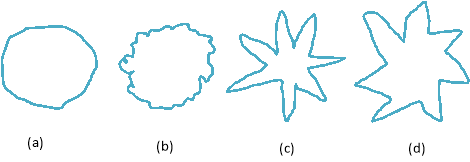From the digital mammogram image, the shape of the benign tumor is round and smooth, but the shape of the malignant tumor is irregular and roughly bounded. This main difference is utilized to categorize the benign tumor and malignant tumor. The following Figure-1 depicts the morphological spectrum of the breast masses frequently seen in digital mammograms. Figure-1:(a). Round Benign (b). Lobulated benign (c). Malignant (d). Malignant Proposed ModelMost of the medical image processing applications used fractal analysis and which is focused in various researches on the digital mammograms. In this study, it is experimenting using the FFT based fractal analysis and classifying the breast lesions. The complete flow of this study is depicted in Figure-2. Figure-2: Overall Flow of the Proposed Approach The structure of this study is described as given below, section-III discussed about the hybrid filter and its applications. Section-IV discussed about the basic information about the fractal analysis method. Section-V described about Fractals based or Fourier Transform method. Section-VI described about our experiment and results. Section-VI provides the conclusion about this study and suggestion for further enhancement work. Hybrid Filter The hybrid filter combines morphological filter with the Gabor filter for removing the noise from the mammogram image to improve the quality of the image. Morphological filter is a non-linear filter work based on the set theory rules and Gaussian filter is a linear filter work based on vectors and both are used to remove noise.The main motto of this hybrid filter is to completely remove various noises occur under different conditions in the image, to improve the performance of the proposed approach. Morphological filter can remove the noise on the contour of the image and Gabor filter remove the noise in the inside of the image. Morphological filter utilized various morphological transform using different structuring elements. In this study also, different morphological transform is tested while experimenting to improve the appearance of the contour. The morphological functions are defined as:— (1) Where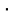denotes the opening operation and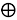denotes the dilation anddenotes the morphological erosion operation. Device’s mechanism introduced two kinds of noise such as coherence and no-coherence noise. The Gaussian noise is represented by statistical noise, having a probability density function, which is called as a Gaussian distribution. The original pixel value in the image is changed from its inventive value by a minute amount in the Gaussian noise. Due to the central bound theorem, Gaussian distribution is generally can provide a good quality representation. The probability density function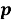of a Gaussian random variable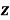is given by: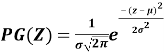— (2) Alternatively, a process is Gaussian if and only if for every finite set of indicesin the index set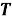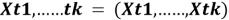— (3) It is a multivariate Gaussian random variable. The Gaussian property can be formulated by using the features functions of random variables as: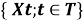, such that— (4) The hybrid filter can effectively remove all the noise in the mammogram image which can provide more accuracy in classification. Fractal Analysis There are various fractal analysis techniques are existing but most of the techniques follow power law basics. In the exisiting work , tumor growth was studied with the help of a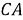model which says that the tumor is a rising tissues. Mathematical model and numerical simulations of this model were examined to obtain the macroscopic dynamics of the tumor growth. It experimented and well known that the growth of the tumor is proportional to the time  suggested from power law. It is also can be simulated using a one-dimensional (1D) CA model, shows the linear growth of the entire cells. From this, it is observed that in both the 1D and 2D cases, tumor diameter grows linearly according in terms of time.The dimension of the fractal model is estimated using various techniques such as sandboxes, bounding-box, Fourier spectrum and so on. When applying these techniques, the scaling relationships of the cells are obtained according to a power law relationship. The basic geometric objects can be understood by the Euclidean objects as lines, planes and circles. All the objects do not resemble the Euclidean objects. By utilizing the fractal geometry, it is easy to create models for nature objects and which can provide a better definition in various conditions. Mandelbrot  introduced the first fractal theory. The unique difference among the Euclidean and fractal geometry is the self similarity denoting by un-uniform scaling. The variance of the shape of the objects continuously varying in increasing or decreasing the size of the objects. It is clear that one of the problems in scaling is texture, and describing the texture also depends on scaling. Hence, this problem can be overcome by the fractal geometry of texture. The definition of Hausdroff-Besicovitch of the fractal dimension is described using the following equation (5).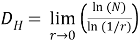— (5) Whereis the self similar pieces 1/r is the magnificent factor. Since, the fractal dimension indicates the surface roughness, people always use the texture as fine, coarse, gained and smooth etc. Mostly the fractal dimension of an image can be estimated by the bounding-box, fractal Brownian motion and fractal interpolation method. In this study, the fractal of filtered contour of the breast tumors are analyzed and tested using FFT based methods. Fractal based Fourier Transform In this study, it is adopted that Fourier fractal methodology is used for classifying the tumors. The filtered contour is taken and fed as input for testing. The growth of the tumor is randomness and it is in certain degrees, complex irregular in shape. So that, the fractal analysis can give a good measure in order to measure the complex patterns than the traditional Euclidean geometry. In this study, the fractal dimension is measures using Fourier transform method. In our experiment the radical magnitude accusations are calculated and plot in the form of log-log magnitude plot for classifying the tumors. From the centroid to all directions, the magnitude variation is measured to compute the magnitude accumulation testing. The Fourier transforms and phase angle calculations are obtained using Equations (3) and 4) respectively. The filtered contour is defined in X-axis with M – mean value and it can be implemented as: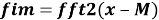— (6)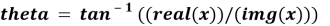— (7) In this study, the fractal dimension of the breast tumor is calculated according to the average slop variations of log-log magnitude plot. Also the log-log plot can be drawn among the magnitude accumulation in entire radial components and number radial components of the respective input images. The accurate and absolute values of average variations are more for malignant tumor than the benign tumors. Experiment and Results To experiment Fractal based fourier transform analysis method the matlab software is choosen to implement, due to its capability in image processing. In this study, it is considered that some of the available contours are the input for the experiment. For pre-processing the image, to remove, the higher order frequency components are taken as artifacts, the input contours are applied into hybrid filters. The result of the hybrid filter is separated into small segments with dissimilar radius length by dividing the contour uniformly in all directions. In our experiment, the contour is divided into 24 equal parts. Then the fractal dimension method using FFT is applied to extract shape variables in each segment. In our experiment, five contours are considered as the input, at five, three contours are the type of malignancy and the remaining contours belongs to the type of benign. The following Table-1 depicts the log-log magnitude plot and absolute values of the slop variations in terms of respective contour. After filtering the input contour it is divided into 24 segments in all the directions with equal radial distance and then the magnitude variation of all the directions is counted and accumulated for all the radial components. It is well known that the malignant tumor has more variations than the benign tumors. According to the accumulations of the magnitude variations the malignant tumors are having more variations than the benign masses. The fractal dimension is calculated using the log-log plot drawing method between the accumulations of magnitude variations and the number of radial components. The absolute value [a threshold value used for decision making] is drawn in the log-log plot to compute the average variation of the magnitude. To provide difference the slop, the colors used to plot are different. The absolute value of the average slop variation according to the threshold is used to classify the tumors. In this experiment, a threshold value is used for decision making, for tumor classification. The absolute value of the average slop difference is high for malignant and for the same value it is less for benign. From this scenario, it is observed that, the variations of magnitude accumulations in terms of number of radius are more for malignant tumor and small for a benign tumor. Contour Image Log-Log Plot Absolute value of the Average plot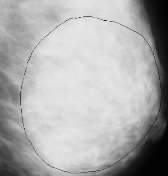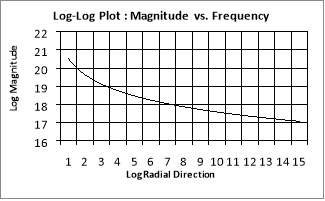0.9802 B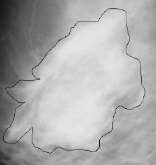1.0954 M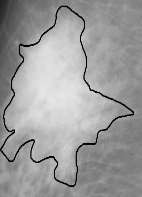1.01 M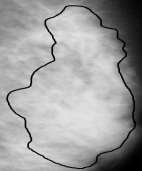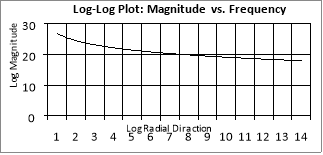2.198 B-M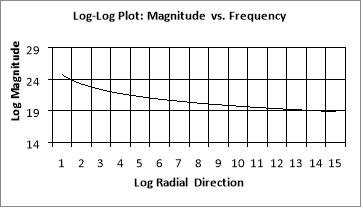2.543 M             Table-1: Fractal Analysis based on FFT Tumor classification In most of the cases the average slope values are greater than the threshold values for our test images used in the experiment. Originally the test image 1 is the benign and the other images are the malignant images. Generally Image 4 is malignant image, but according to the average slop variations and threshold values it is defined as benign. In this paper, we experiment with 25 images[ but in table-1 only five images and their results are displayed]. Out of 25 it correctly classifies 23 images. From this experiment 92% of success rate. Conclusion and Future Enhancement The FFT based fractal analysis method is easy to implement and classify the tumors based on the shape of the tumors. In this study, from the experiment, FFT based fractal analysis method achieved 92% of the classification accuracy. Since this study can provide better results than the existing approaches. The accuracy can be improved in the future enhancement of this study. FFT based fractal analysis is one of the easiest methods and best software for doctors to prescribing the tumor and understand the tumor shapes accurately and fast. References .James W. Baish and Rakesh K. Jain, “Fractals and Cancer”, American Association for Cancer Research -2000. . Breast cancer facts and figures http://www.breastcancer.org/. . Syed Abdaheer.M and Ekram Khan, “Shape Based Classification Of Breast Tumors Using Fractal Analysis”, IEEE-2009.  Us-Krasovec M, Erzen J, Zganec M et. Al, “Malignancy associated changes in epithelial cells of buccal mucosa: a potential cancer detection test”, Anal Quant Cytol Histol. 27(5): 254-62, Oct. 2005.  Andrushkiw R.I., Boroday N.V., Klyushin D.A., “Petunin Yu.A. Computer-aided cytogenetic method of cancer diagnosis”, New York: Nova Science Publishers, 2007. . Pelton K, Freeman MR, Solomon KR, “Cholesterol and prostate cancer”, Curr Opin Pharmacol 12: 751 – 759-2012. . Zadra G, Photopoulos C, Loda M, “The fat side of prostate cancer”, Biochim Biophys Acta 1831: 1518 – 1532-2013. . Ramis-Conde I, Drasdo D, Anderson AR, Chaplain MA, “Modeling the inuence of the e-cadherin-beta-catenin pathway in cancer cell invasion: a multiscale approach”, Biophys J 1: 155–165-2008. . Rietman EA, Friesen DE, Hahnfeldt P, Gatenby R, Hlatky L, et al, “An integrated multidisciplinary model describing initiation of cancer and the warburg hypothesis”, Theor Biol Med Model 10-2010. . Gillies RJ, Verduzco D, Gatenby RA, “Evolutionary dynamics of carcinogenesis and why targeted therapy does not work”, Nat Rev Cancer 12: 2012. . Preziosi L, Vitale G, “A multiphase model of tumor and tissue growth including cell adhesion and plastic reorganization”, Math Models Methods Appl 21: 1901–32-2011. . Bellomo N, Delitala M, “From the mathematical kinetic, and stochastic game theory to modelling mutations, onset, progression and immune competition of cancer cells”, Physics of Life Reviews 5: 183–206-2008. . Anderson AR, Quaranta V, “Integrative mathematical oncology”, Nat Rev Cancer 8: 227–34-2008. . Anderson AR, Weaver AM, Cummings PT, Quaranta V, “Tumor morphology and phenotypic evolution driven by selective pressure from the microenvironment”, Cell 127: 905–15-2008 . Agur Z, Vuk-Pavlovic´ S, “Mathematical modeling in immunotherapy of cancer: personalizing clinical trials”, Mol Ther 20: 2012. . Silva AS, Gatenby RA,”A theoretical quantitative model for evolution of cancer chemotherapy resistance”, Biol Direct 5-2010. . Vainstein, V., Kirnasovsky, O.U., Kogan, Y., Agur, Z.: “Strategies for cancer stem cell elimination: Insights from mathematical modeling”. J. Theor. Biol. 298, 32-41 (2012).

## Order a Unique Copy of this Paper

Essay Creek is an academic writing service provided to you by, a London-based company.

• Experience
Helping students successfully for 11 years.
• Confidentiality & Security
Be sure your information will be kept confidential due to our secure service.
• Quality & Reliability
8.5 out of 10 average quality score according to our customers' feedback. 97.45% of orders delivered on time.
• Versatility
478 active writers in 68 disciplines.
• 100% money back guarantee
You can always request a refund if you are not satisfied with the result.

Our team of writers is comprised of people with necessary academic writing skills and experience in various fields of study.

• Skilled writers only
We carefully choose writers to employ, paying attention to their skills and abilities.
• Competence
Your order will be assigned to a competent writer who specializes in your field of study.
• In-depth knowledge
Our writers know both peculiarities of academic writing and paper formatting rules.
• Motivation
We keep updated on results our writers show, motivating them to constantly improve their performance.